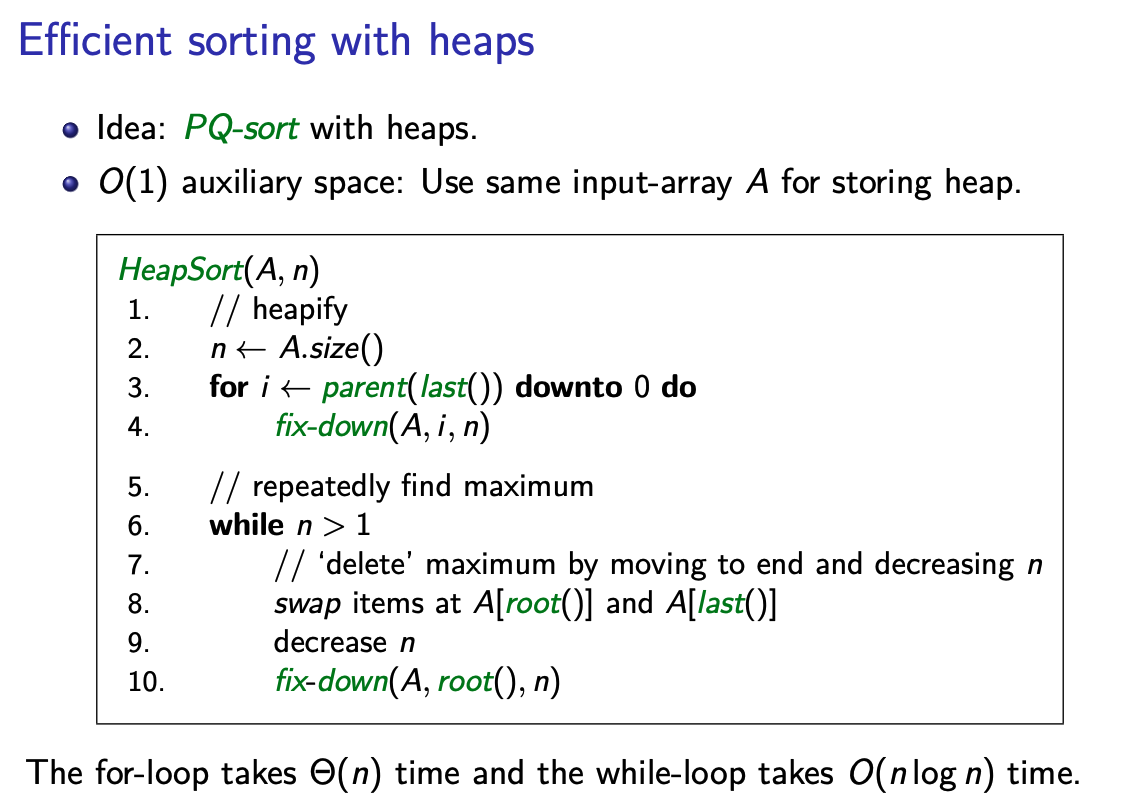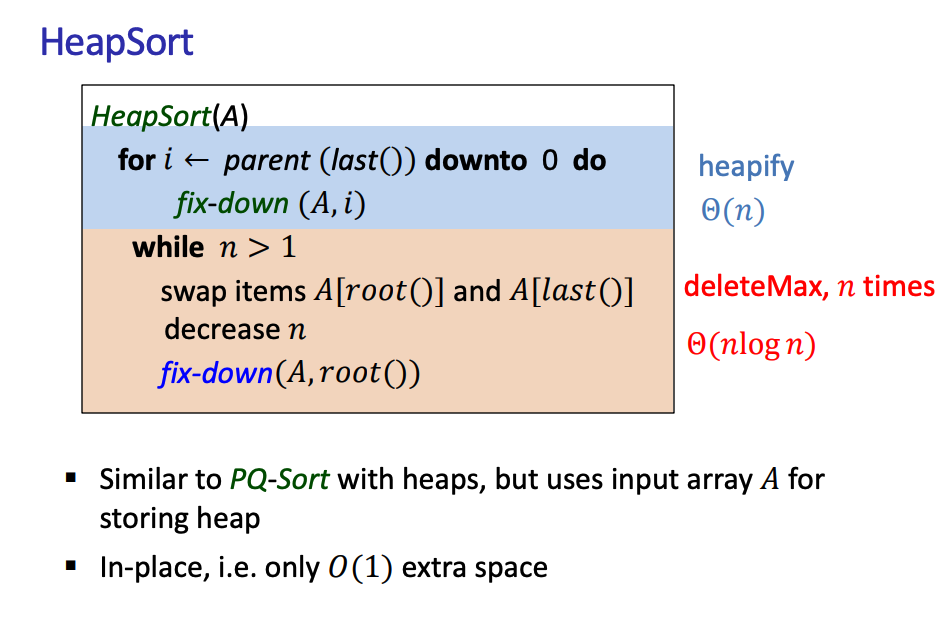Sorting Algorithms

# Heap Sort §

Heap sort is a comparison-based sorting technique based on the Binary Heap. It is similar to selection sort where we first find the minimum element and place the minimum element at the beginning. We repeat the same process for the remaining elements.

Make sure to understand the Heapify operation.Here’s another way to look at heap sort, as explained by teacher OlgaThe advantage is that memory complexity of .

https://github.com/Gongsta/420-LCW-MS-Programming-Techniques-and-Applications/blob/main/l4code/heapsort.py

def _lower(data, k, n):
'''This is the critical step of the heap creation
process. The idea is that, after a deletion, we have
to demote a key that now violates the heap principle.'''

j = k * 2 + 1

while j < n:
if j < n - 1:
if data[j] < data[j + 1]:
j += 1 # right child

if data[k] >= data[j]:
break

data[j], data[k] = data[k], data[j]
k = j
j = k * 2 + 1

def heapsort(data):
'''
heap sort an array using a priority queue.
This implements an in-place, N log N sort.
'''

# Create a priority queue from the initial array.
n = len(data) - 1
i = (n - 1) // 2
while i >= 0:
_lower(data, i, n + 1)
i -= 1

# Now we essentially sort the array by taking the maximum
# element, swapping it to the back of the array, and then
# 'sinking' the old last element to its proper position.

while n >= 0:
data, data[n] = data[n], data
_lower(data, 0, n)
n -= 1